Volume Integrals over n-Dimensional Ellipsoids by Stanislav Sýkora, Extra Byte, Via R.Sanzio 22C, Castano Primo, Italy 20022 in Stan's Library, Ed.S.Sykora, Vol.I. First release April 30, 2005 Permalink via DOI:  10.3247/SL1Math05.001
 Other math articles | Stan's Library Stan's LINKS and  Courses | Stan's HUB
 Abstract In a paper  on quantum theory, the Autor had to find a general formula for the surfaces of spheres in n-dimensional complex spaces. Since the focus was then strictly physical, the math has been reduced to a bare minimum. This Note is the first one of a series exploring the mathematical aspects of the topic in more detail and in a step-by-step manner. It focuses on the evaluation of integrals of products of powers of Cartesian vector components over the volumes of n-dimensional spheres and ellipsoids in real, n-dimensional Euclidean spaces. The integrals tackled here are expressed by means explicit formulae which merit attention on their own. Next Note in this series: Surface Integrals over n-Dimensional Spheres.

1. Introduction

We will focus our attention on certain classes of volume integrals over n-dimensional real ellipsoids which, as we shall see, can be integrated explicitly. For the moment we postpone the evaluation of surface integrals because they are much more difficult to handle. In the simple case of two-dimensions, for example, the area of an elipsis is given by the explicit formula πab (a,b being the two semiaxes), while its circumference involves transcendental elliptic integrals. There is a relationship between the two categories, however, so the effort needed to analyse volume integrals will not be lost.

Let Rn denote the n-dimensional Euclidean space. The position vector r and its norm r = |r| of a point in Rn and its norm r can be written as

(1)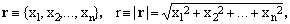where the x's are its Cartesian coordinates. We shall often use n-tuples of non-negative real exponents

(2)which, however, are not to be intended as elements of Rn. The shorthand will be exploited in conventional expressions of the type

(3)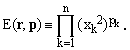(4)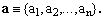will be used to define ellipsoid volumes V(a) in Rn by means of the condition

(5)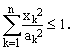This implies that the ellipsoid axes are aligned with the Cartesian axes and that, for the sake of uniqueness, all the a's should be non-negative. We shall be interested in the evaluation and analysis of the following integrals over ellipsoid volumes V(a):

(6)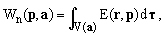where dτ is the volume element.

2. Evaluation of the integrals

In Cartesian coordinates, the volume element dτ is given by

(7)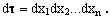Applying the coordinates-scaling transformation

(8)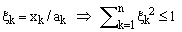one obtains

(9)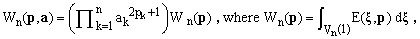with Vn(R) denoting the interior of an n-dimensional sphere of radius R (in this case, R=1).

To compute the last integral in (9), we can proceed iteratively, reducing the dimensionality of the problem by 1 in each step. The setting

(10)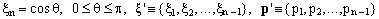corresponds to a cut of the n-dimensional sphere at a value of the last coordinate given by the cosine of the polar angle θ. Considering the inequality in (8), we see that the cut is an (n-1)-dimensional sphere of radius R = sinθ, so that

(11)An (n-1)-dimensional sphere of radius R is an ellipsoid whose semi-axes set a' has all components equal to R. Hence, using Eq.(9),

(12)Substituting the last equation into (11), one obtains

(13)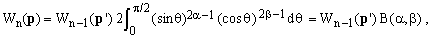where B(α,β) is the beta function [4,5] and

(14)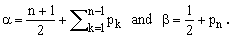The beta function can be expressed by means of the gamma function [4,5] which leads to

(15)The starting term (for n=1) in the recurrence (13) is easily determined by explicit integration (it can be also shown that the recurrence is compatible with the formal assumption according to which a zero-dimensional unit sphere has volume 1). Using the recurrence and applying repetitively Eq.(15), it is straightforward to determine that

(16)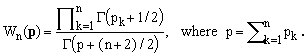Combining this with Eq.(9) and taking advantage of the fact that Γ(z+1) = zΓ(z), we finally obtain the following closed-form expression for the desired integral over an ellipsoidal volume

(17)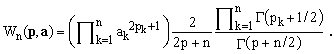3. Admissible values of the exponents (p's)

In equations (3), (6) and (11) we have made it clear that an individual Cartesian coordinate xk get first squared and only afterwards elevated to pk (this fact is essential also in the passage from II.5 to II.7). Consequently, the values of pk are not limited to natural integers and zero but may assume any real value greater than -1/2 , a bound due to the fact that the α and β in Eq.(13) must be positive for the beta function definition to hold.

4. Special cases

Setting all the p's equal to ν/2, one obtains the following formula, valid for any ν>-1:

(18)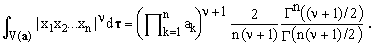In particular, setting ν = 1 and taking into account that Γ(1) = 1, we obtain the interesting formula

(19)Notice that for n-dimensional spheres of unit radius, the right-hand side of this identity reduces to 1/n!.

When only one of the p's equals ν/2 and all the others are zero, Eq.(17) yields the identity, again valid for any ν>-1:

(20)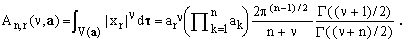For the important cases of ν=1 and ν=2, this gives

(21)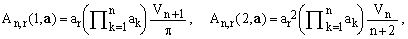where

(22)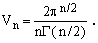Finally, summing over all indices r, the latter identity yields another interesting formula:

(23)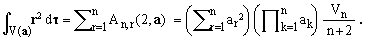5. Volumes of n-dimensional ellipsoids and spheres

When all the p's are zero, Eq.(17) gives simply the volume of an ellipsoid whose main axes are specified by the set a. Explicitly, this turns out to be

(24)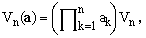showing that the the numbers Vn of Eq.(22) are the volumes of n-dimensional spheres of unit radius. Their values can be easily calculated by means of the recurrence

(25)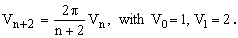The volume of an n-dimensional ellipsoid has the dimension of the n-th power of length. This coincides with the popular notion of "volume" only in the case of n=3 but its extension to any n is quite obvious. In particular, for n=2 it coincides with the common concept of "area" of an ellipse and for n=1 with the "length" of a 1D-interval. The value of 1 for n=0, implicit in the above recurrence relations, might be considered as the cardinality of a set of a single 0D point.

Volumes and other volume integrals for n-dimensional spheres with unit radius

 Dim Volume Volume integral of: symbolic numeric |x1| x12 r2 |x1x2| x12x22 or x14/3 r4 1 2 2.000000.. 1 2/3 2/3 - - 2/5 2 π 3.141592.. 4/3 π/4 π/2 1/2 π/24 π/3 3 4π/3 4.188790.. π/2 4π/15 4π/5 8/15 4π/105 4π/7 4 π2/2 4.934802.. 8π/15 π2/12 π2/3 π/6 π2/96 π2/4 5 8π2/15 5.263789.. π2/6 8π2/105 8π2/21 16π/105 8π2/945 8π2/27 6 π3/6 5.167712.. 16π2/105 π3/48 π3/8 π2/24 π3/480 π3/10 7 16π3/105 4.724765.. π3/24 16π3/945 16π3/135 32π2/945 16π3/10395 16π3/165 8 π4/24 4.058712.. 32π3/945 π4/240 π4/30 π3/120 π4/2880 π4/36 9 32π4/945 3.298508.. π4/120 32π4/10395 32π4/1155 64π3/10395 32π4/135135 32π4/1365 10 π5/120 2.550164.. 64π4/10395 π5/1440 π5/144 π4/720 π5/20160 π5/168

6. Asymptotic behaviorEquation (22) for the volume of an n-dimensional sphere of unit radius can be rewritten in terms of the following function ν(x):

(26)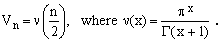The graph of ν(x) is shown on the right. It is evident that it passes through a maximum and then, for x growing to infinity, decays to zero. The rate of the decay for large values of n can be estimated using the Stirling approximation [4,5] for Γ(x), but the result is not particularly enlighting. What it shows is the validity of the following limit

(27)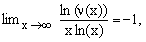indicating that, for very large x, the decay is dominated by a factor of the form O[x-x].

 References Sykora S., Quantum Theory and the Bayesian Inference Problems, J.Statistical Phys. 11, 17 (1974). Abstract. Sykora S., Surface Integrals over n-Dimensional Spheres, in Stan's Library, Ed.S.Sykora, Vol I, 2005. Sykora S., Volume Integrals over n-Dimensional Ellipsoids, in Stan's Library, Ed.S.Sykora, Vol I, 2005. (URL of this Note). Abramowitz M., Stegun A., Editors, Handbook of Mathematical Functions, National Bureau of Standards 1964, Dover Publications, New York 1972. John Wiley & Sons, Reprint edition 1993. Arfken G.B., Weber H., Mathematical Methods for Physicists, Academic Press, 5th edition 2000.
 TOP | Other math articles | Stan's Library Stan's LINKS and  Courses | Stan's HUB | TOP